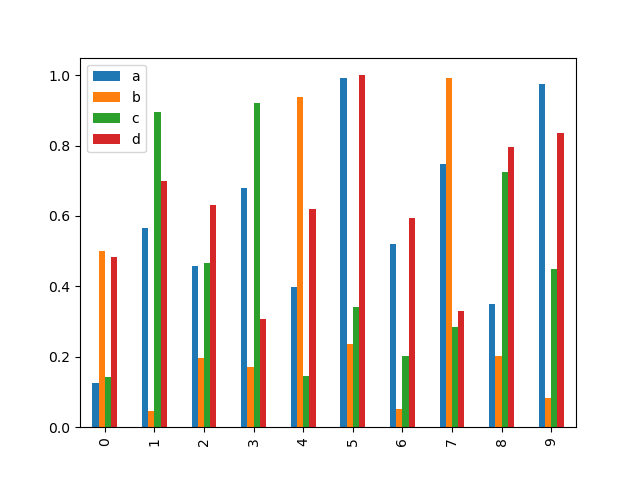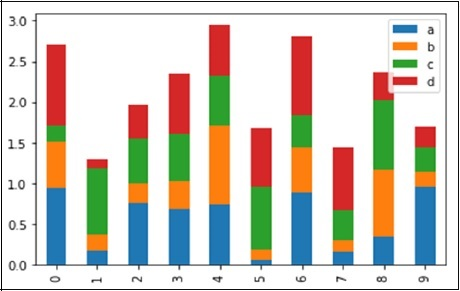python list append multiple times

## Pandas Built in Data Visualization pandas to excel Tutorial

Pandas Built in Data Visualization Tutorial:
Want to learn Pandas Built in Data Visualization?
Welcome to my python crash course blog post.In the previous blog we see Data Visualization with Matplotlib and seaborn,In this lecture we will learn about pandas built-in capabilities for data visualization! In the next blog we will see what is pandas to excel ? It’s built-off of matplotlib,
but it baked into pandas for easier usage!
Let’s take a look!
import numpy as np
import pandas as pd
%matplotlib inline
There are some fake data csv files you can read in as dataframes:
Also see : Numpy in python

## Style Sheets

Matplotlib has style sheets you can use to make your plots look a little nicer. These style sheets include plot_bmh,plot_fivethirtyeight,plot_ggplot and more. They basically create a set of style rules that your plot follow. I recommend using them, they make all your plots have the same look and feel more professional. You can even create your own if you want your company’s plots to all have the same look (it is a bit tedious to create on though).
Here is how to use them

# Plot Types

There are several plots types built-in to pandas, most of them statistical plot in nature:
1. df.plot.area
2. df.plot.barh
3. df.plot.density
4. df.plot.hist
5. df.plot.line
6. df.plot.scatter
7. df.plot.bar
8. df.plot.box
9. df.plot.hexbin
10. df.plot.kde
11. df.plot.piepandas built in data visualization in python easy example fig 01) Pandas Built in Data Visualization pandas to excel  Tutorial

You can use c to color based off another column value Use cmap to indicate colormap to use.
For all the colormaps, check out: http://matplotlib.org/users/colormaps.html
That’s it! Hopefully you can see why this method of plotting will be a lot easier to use than full-on matplotlib, it balances ease of use with control over the figure. A lot of the plot calls also accept additional arguments of their parent matplotlib plt. call.
Next we will learn about seaborn, which is a statistical visualization library designed to work with pandas dataframes well.
Before that though, we’ll have a quick exercise for you! so Next blog post on pandas to excel.

## Bar Plot

Let us now see what a Bar Plots is by creating one. A bar plots can be created in the following way −
`import pandas as pdimport numpy as npdf = pd.DataFrame(np.random.rand(10,4),columns=['a','b','c','d')df.plot.bar()`
Its output is as follows −pandas built in data visualization in python easy example
To produce a stacked bar plots, pass stacked=True −
`import pandas as pddf = pd.DataFrame(np.random.rand(10,4),columns=['a','b','c','d')df.plot.bar(stacked=True)`
Its output is as follows −pandas built in data visualization in python easy example

FOR DETAILED  OPEN DRIVE LINK: